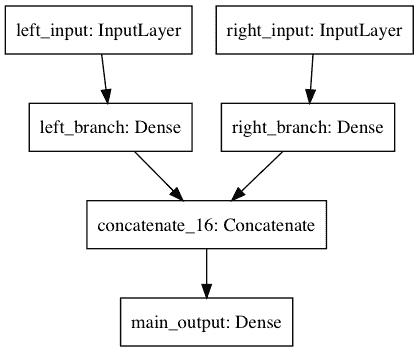# Keras Functional API

## Recall: All models (layers) are callables

``````from keras.layers import Input, Dense
from keras.models import Model

# this returns a tensor
inputs = Input(shape=(784,))

# a layer instance is callable on a tensor, and returns a tensor
x = Dense(64, activation='relu')(inputs)
x = Dense(64, activation='relu')(x)
predictions = Dense(10, activation='softmax')(x)

# this creates a model that includes
# the Input layer and three Dense layers
model = Model(inputs=inputs, outputs=predictions)
model.compile(optimizer='rmsprop',
loss='categorical_crossentropy',
metrics=['accuracy'])
model.fit(data, labels)  # starts training
``````

# Multi-Input Networks

## Keras Merge Layer

Here's a good use case for the functional API: models with multiple inputs and outputs.

The functional API makes it easy to manipulate a large number of intertwined datastreams.

Let's consider the following model.

``````from keras.layers import Dense, Input
from keras.models import Model
from keras.layers.merge import concatenate

left_input = Input(shape=(784, ), name='left_input')
left_branch = Dense(32, input_dim=784, name='left_branch')(left_input)

right_input = Input(shape=(784,), name='right_input')
right_branch = Dense(32, input_dim=784, name='right_branch')(right_input)

x = concatenate([left_branch, right_branch])
predictions = Dense(10, activation='softmax', name='main_output')(x)

model = Model(inputs=[left_input, right_input], outputs=predictions)
``````

Resulting Model will look like the following network:Such a two-branch model can then be trained via e.g.:

``````model.compile(optimizer='rmsprop', loss='categorical_crossentropy', metrics=['accuracy'])
model.fit([input_data_1, input_data_2], targets)  # we pass one data array per model input
``````

## Try yourself

#### Step 1: Get Data - MNIST

``````# let's load MNIST data as we did in the exercise on MNIST with FC Nets
``````
``````# %load ../solutions/sol_821.py
``````

#### Step 2: Create the Multi-Input Network

``````## try yourself
``````
``````## `evaluate` the model on test data
``````

Keras supports different Merge strategies:

• `add`: element-wise sum
• `concatenate`: tensor concatenation. You can specify the concatenation axis via the argument concat_axis.
• `multiply`: element-wise multiplication
• `average`: tensor average
• `maximum`: element-wise maximum of the inputs.
• `dot`: dot product. You can specify which axes to reduce along via the argument dot_axes. You can also specify applying any normalisation. In that case, the output of the dot product is the cosine proximity between the two samples.

You can also pass a function as the mode argument, allowing for arbitrary transformations:

``````merged = Merge([left_branch, right_branch], mode=lambda x: x - x)
``````

# Even more interesting

Here's a good use case for the functional API: models with multiple inputs and outputs.

The functional API makes it easy to manipulate a large number of intertwined datastreams.

Let's consider the following model (from: https://keras.io/getting-started/functional-api-guide/ )

## Problem and Data

The main input to the model will be the headline itself, as a sequence of words, but to spice things up, our model will also have an auxiliary input, receiving extra data such as the time of day when the headline was posted, etc.

The model will also be supervised via two loss functions.

Using the main loss function earlier in a model is a good regularization mechanism for deep models.``````from keras.layers import Input, Embedding, LSTM, Dense
from keras.models import Model

# Headline input: meant to receive sequences of 100 integers, between 1 and 10000.
# Note that we can name any layer by passing it a "name" argument.
main_input = Input(shape=(100,), dtype='int32', name='main_input')

# This embedding layer will encode the input sequence
# into a sequence of dense 512-dimensional vectors.
x = Embedding(output_dim=512, input_dim=10000, input_length=100)(main_input)

# A LSTM will transform the vector sequence into a single vector,
# containing information about the entire sequence
lstm_out = LSTM(32)(x)
``````
``````Using TensorFlow backend.
``````

Here we insert the auxiliary loss, allowing the LSTM and Embedding layer to be trained smoothly even though the main loss will be much higher in the model.

``````auxiliary_output = Dense(1, activation='sigmoid', name='aux_output')(lstm_out)
``````

At this point, we feed into the model our auxiliary input data by concatenating it with the LSTM output:

``````from keras.layers import concatenate

auxiliary_input = Input(shape=(5,), name='aux_input')
x = concatenate([lstm_out, auxiliary_input])

# We stack a deep densely-connected network on top
x = Dense(64, activation='relu')(x)
x = Dense(64, activation='relu')(x)
x = Dense(64, activation='relu')(x)

# And finally we add the main logistic regression layer
main_output = Dense(1, activation='sigmoid', name='main_output')(x)
``````

### Model Definition

``````model = Model(inputs=[main_input, auxiliary_input], outputs=[main_output, auxiliary_output])
``````

We compile the model and assign a weight of 0.2 to the auxiliary loss.

To specify different loss_weights or loss for each different output, you can use a list or a dictionary. Here we pass a single loss as the loss argument, so the same loss will be used on all outputs.

#### Note:

Since our inputs and outputs are named (we passed them a "name" argument), We can compile&fit the model via:

``````model.compile(optimizer='rmsprop',
loss={'main_output': 'binary_crossentropy', 'aux_output': 'binary_crossentropy'},
loss_weights={'main_output': 1., 'aux_output': 0.2})
``````
``````
# And trained it via: